# 1 5 Linear Equations Mr Anderson Pre Calculus

• Slides: 16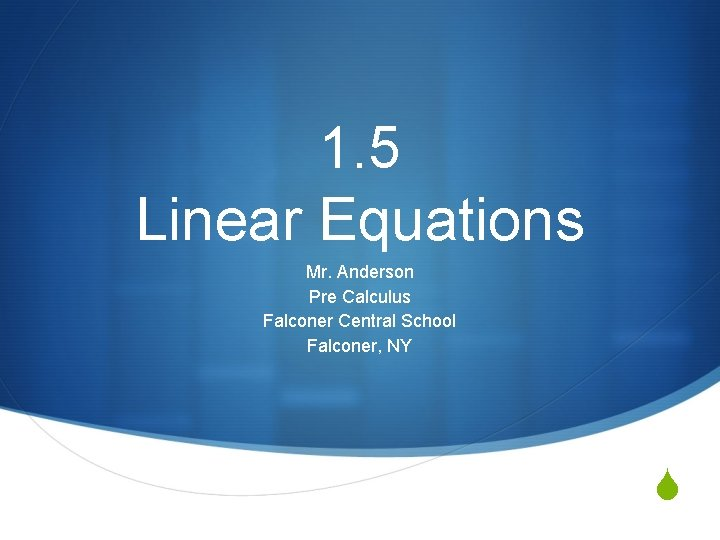1. 5 Linear Equations Mr. Anderson Pre Calculus Falconer Central School Falconer, NY S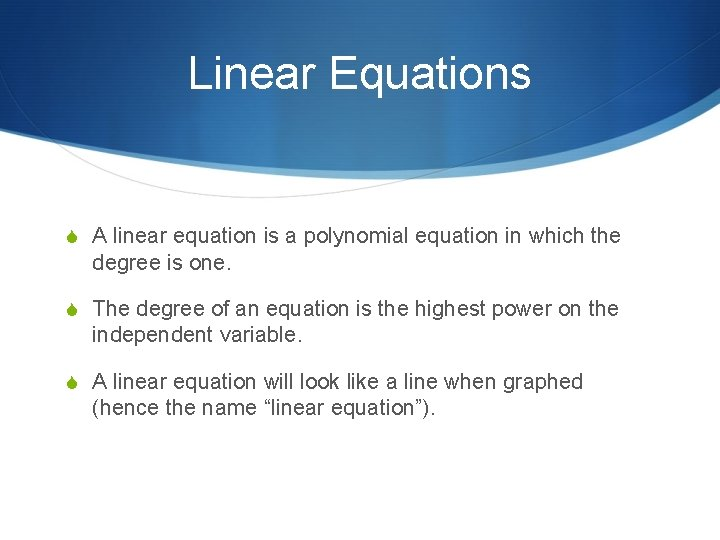Linear Equations S A linear equation is a polynomial equation in which the degree is one. S The degree of an equation is the highest power on the independent variable. S A linear equation will look like a line when graphed (hence the name “linear equation”).A Couple Forms S Explicit – Solved for the dependent variable S Implicit – Solved for the constant S *Note that polynomial equations are typically written in explicit forms.Slope – Intercept Form S This is the form that we are used to. It requires a slope, and a y-intercept (hence the name).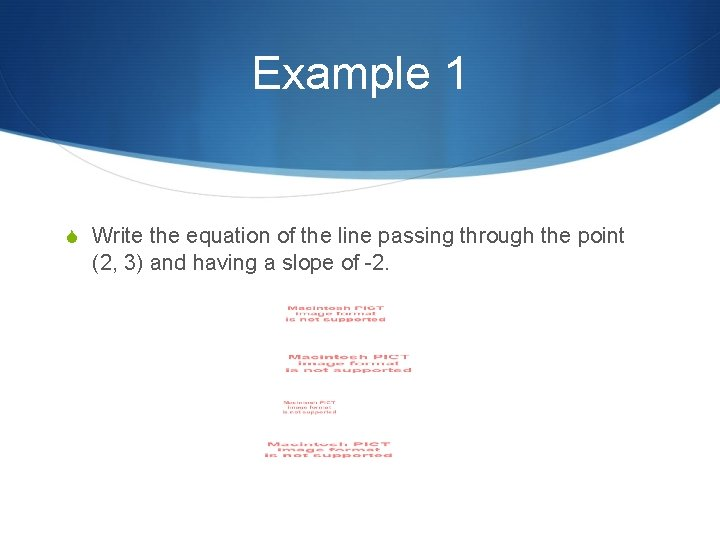Example 1 S Write the equation of the line passing through the point (2, 3) and having a slope of -2.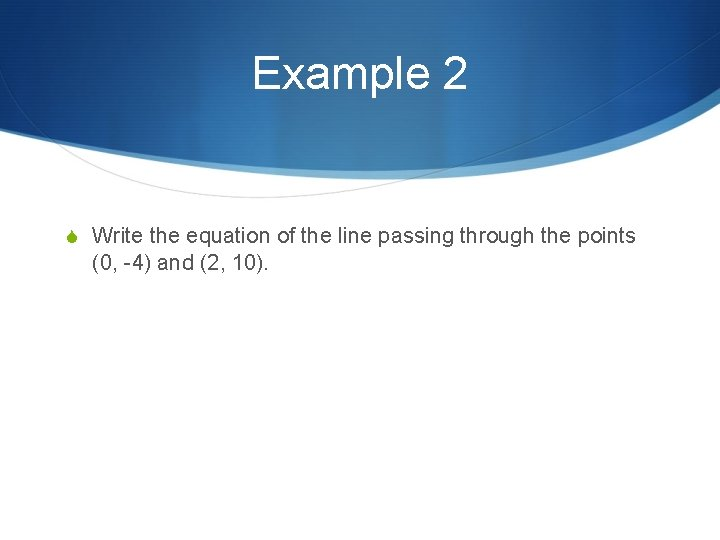Example 2 S Write the equation of the line passing through the points (0, -4) and (2, 10).1. 6 Parallel and Perpendicular Lines Mr. Anderson Pre Calculus Falconer Central School Falconer, NY S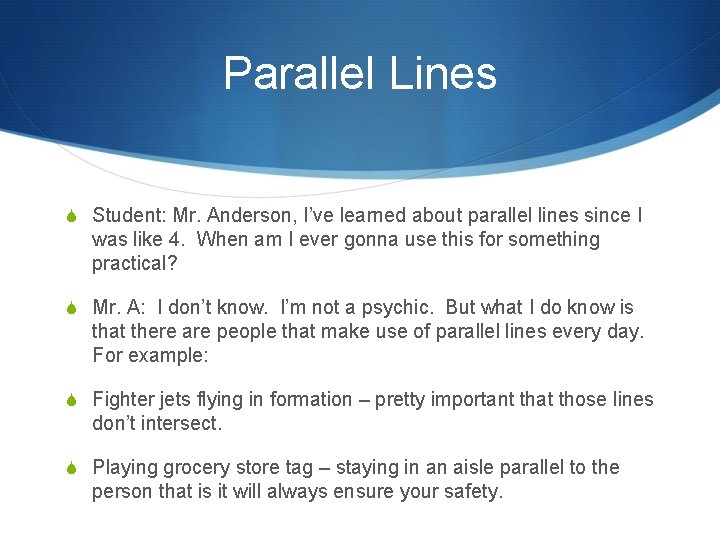Parallel Lines S Student: Mr. Anderson, I’ve learned about parallel lines since I was like 4. When am I ever gonna use this for something practical? S Mr. A: I don’t know. I’m not a psychic. But what I do know is that there are people that make use of parallel lines every day. For example: S Fighter jets flying in formation – pretty important that those lines don’t intersect. S Playing grocery store tag – staying in an aisle parallel to the person that is it will always ensure your safety.City Designers S Parallel and perpendicu lar streets maximize efficiency.Railroad Engineers S Very important that these rails don’t intersect.If they do: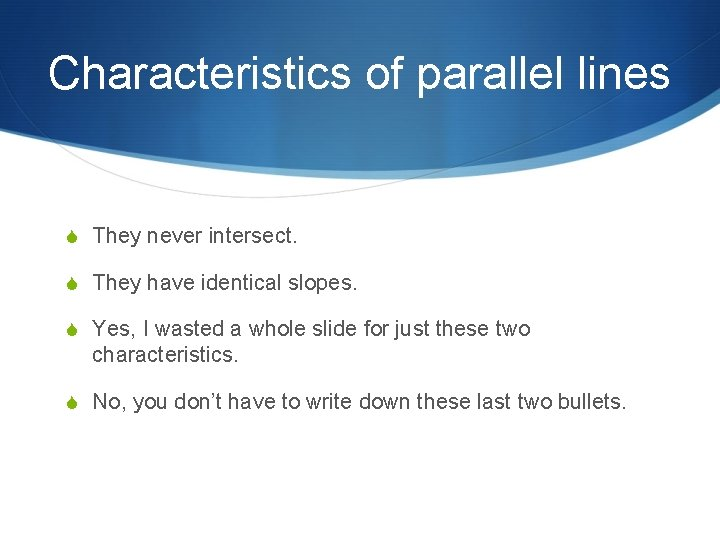Characteristics of parallel lines S They never intersect. S They have identical slopes. S Yes, I wasted a whole slide for just these two characteristics. S No, you don’t have to write down these last two bullets.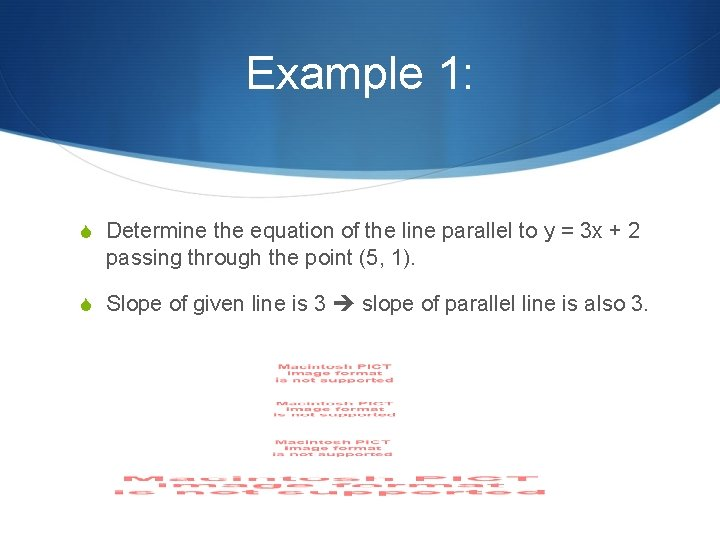Example 1: S Determine the equation of the line parallel to y = 3 x + 2 passing through the point (5, 1). S Slope of given line is 3 slope of parallel line is also 3.Perpendicular Lines S Perpendicular lines always intersect at right angles. S The slopes of perpendicular lines are negative reciprocals of each other. S BTW… if you are upset that you are writing this info down, and you’re offended because you’ve learned this since you were like 4… don’t write it down. I’m happy this is not new to you.Example 2: S Determine the equation of the line passing through the point (-5, 12) and perpendicular to the line y = 4 x. S Slope of given line is 4 slope of perpendicular line is -¼.Practice S Pg. 46 # 17 -28 , 39